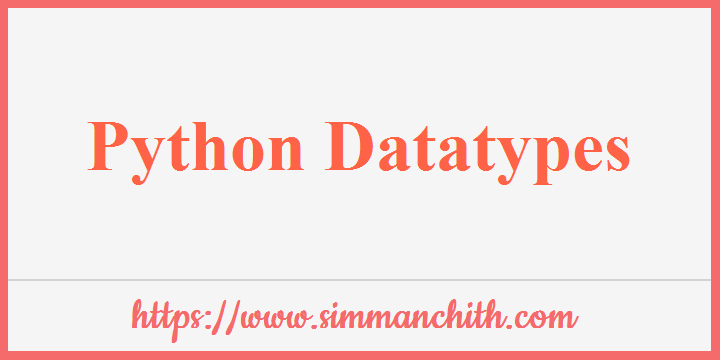# Python Data TypesThe data type is a crucial concept in programming.

Variables can store data of various types, and various types can do various things.

Python includes the following built-in data types by default in these categories :

Text Type : `str`

Numeric Types : `int`, `float`, `complex`

Sequence Types : `list`, `tuple`, `range`

Mapping Type : `dict`

Set Types : `set`, `frozenset`

Boolean Type : `bool`

Binary Types : `bytes`, `bytearray`, `memoryview`

You can also search for these topics, explain python built-in data type, how many built in data types in python, write short on built-in data type in python, built in numeric data type in python, python built in data types, write the names of python built, explain any built-in numeric.

## Getting the Data Type

Use the `type()` function for get the data type of any object :

Example :- The data type of the variable x should be printed :

``````x = 5
print(type(x))``````

Output :-

<class 'int'>

You can also search for these topics, python getting the data type basic, check and change the python get data, The python data type default and declaration, define the python datatype elements, example for python datatypes.

## Setting the Data Type

When you assign a value to a variable in Python, the data type is determined :

Example Data Type
x = "Hello World" str
x = 20 int
x = 20.5 float
x = 1j complex
x = ["apple", "banana", "cherry"] list
x = ("apple", "banana", "cherry") tuple
x = range(6) range
x = {"name" : "John", "age" : 36} dict
x = {"apple", "banana", "cherry"} set
x = frozenset({"apple", "banana", "cherry"}) frozenset
x = True bool
x = b "Hello" bytes
x = bytearray(5) bytearray
x = memoryview(bytes(5)) memoryview

You can also search for these topics, python set data type, example for python setting datatype, generate the setting datatype using python, how to handle the datatype setting, python list the datatype, define the python key value.

## Setting the Specific Data Type

You can use the following constructor functions to specify the data type :

Example Data Type
x = str(Hello World) str
x = int(20) int
x = float(20.5) float
x = complex(1j) complex
x = list(("apple", "banana", "cherry")) list
x = tuple(("apple", "banana", "cherry")) tuple
x = range(6) range
x = dict(name="John", age=36) dict
x = set(("apple", "banana", "cherry")) set
x = frozenset(("apple", "banana", "cherry")) frozenset
x = bool(5) bool
x = bytes(5) bytes
x = bytearray(5) bytearray
x = memoryview(bytes(5)) memoryview
You can also search for these topics, python setting the specific data type elements, get, how to check the Setting Specific Data Type, python in list set the specific datatypes.